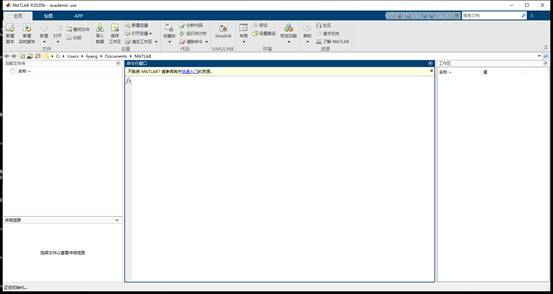• MATLAB-Windows
• MATLAB-MacOS
• MATLAB-Linux

Ø  确保安装MATLAB软件的电脑满足硬件最低配置及系统要求，确认方法请参考：https://cn.mathworks.com/support/sysreq.html

Ø  在安装软件之前，请关闭系统防火墙

Ø  个人版MATLAB需要定期激活，到期后如果用户还是本校师生员工，会自动连接MathWorks服务器激活，用户只需要在出现激活提示时点击确定即可。1. 注册 MathWorks 账户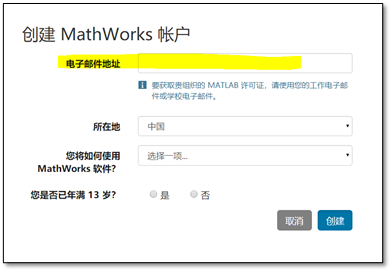2. 下载软件

l  请返回首页下载（推荐）

l  matlab软件校内的下载链接是：Matlab for Windows 64位

l  校外下载新版本或历史版本的MATLAB，请登陆：

3. 启动安装软件

Ø  Windows系统： 解压缩后点击setup.exe（Win10系统可直接用资源管理器打开）

Ø  MacOS系统：双击InstallForMacOSX.app

Ø  Linux系统：执行安装程序命令 ./install

4. 登录到您的 MathWorks 账户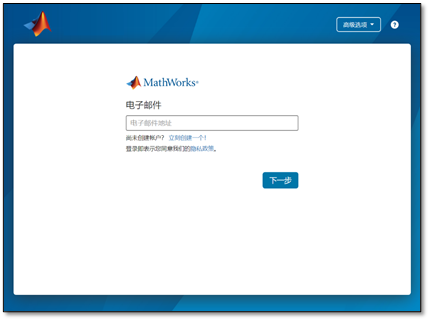5. MathWorks许可协议

6. 选择许可证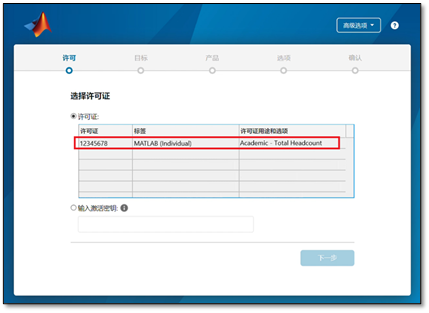7. 选择身份认证选项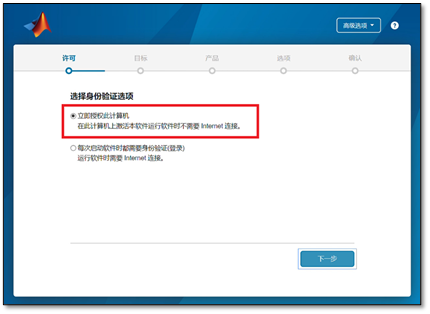8. 确认用户

9. 选择目标文件夹

10. 选择产品

11. 选择选项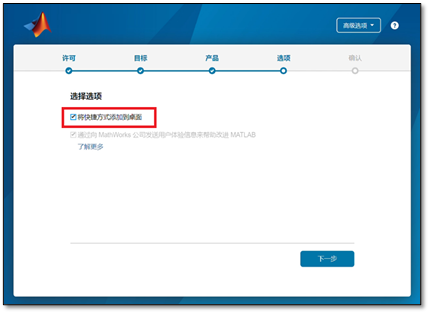12. 确认选择

1. 以管理员身份运行安装程序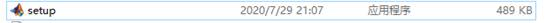2. 高级选项中，选择我有安装密钥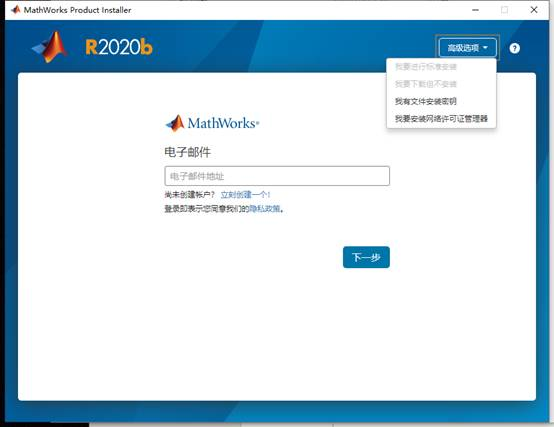3. 接受协议，下一步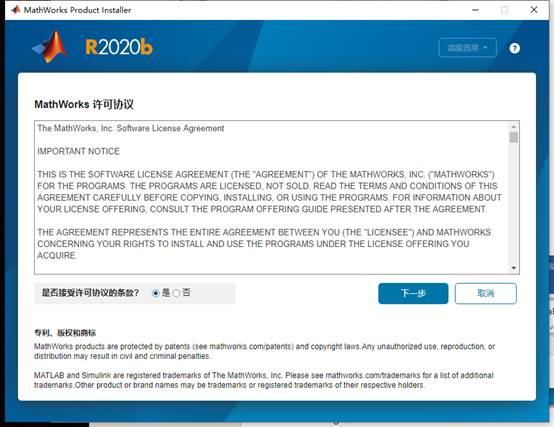4. 输入FIK 下一步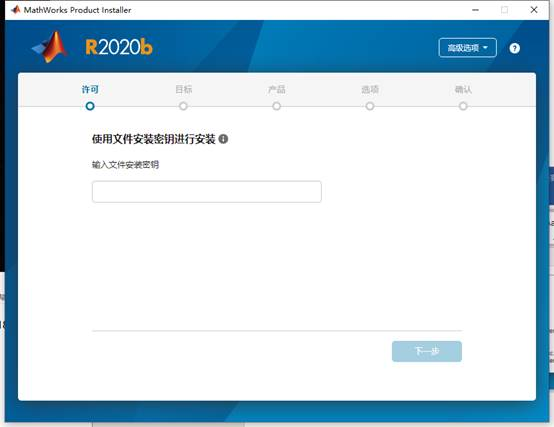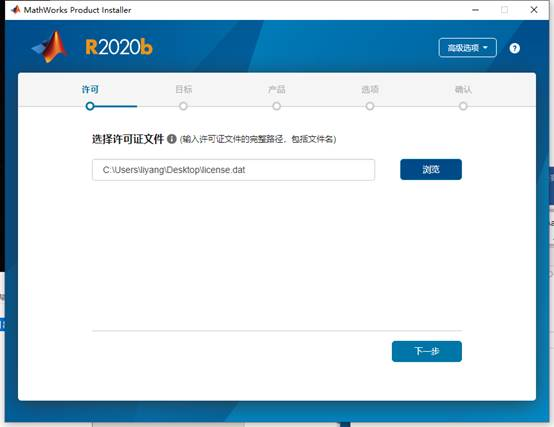6. 选择安装路径 下一步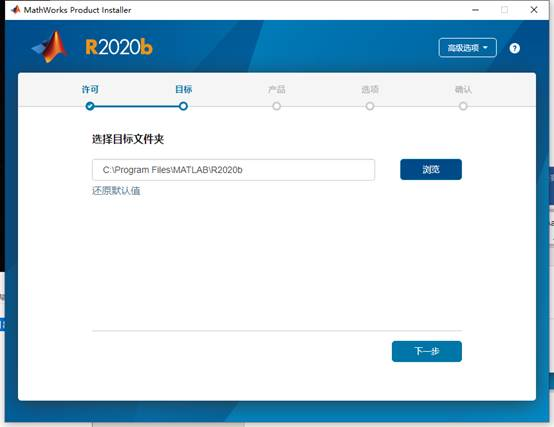7. 全选 下一步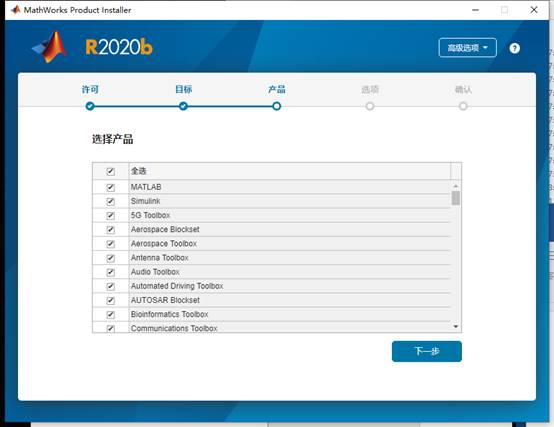8. 创建桌面快捷方式 下一步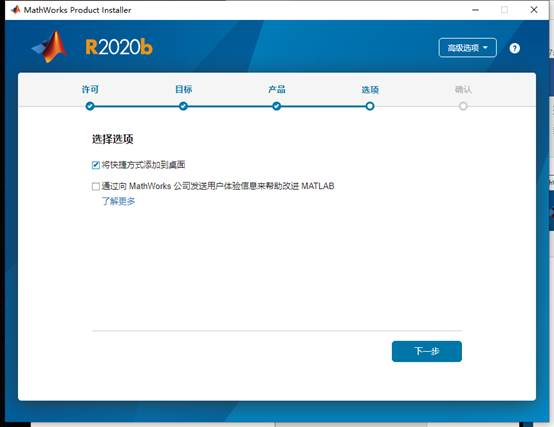9. 开始安装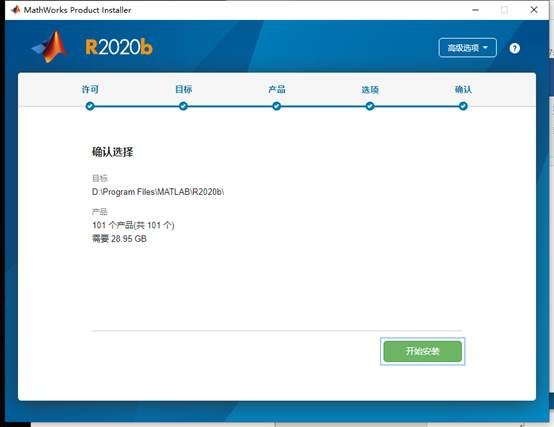10. 安装完成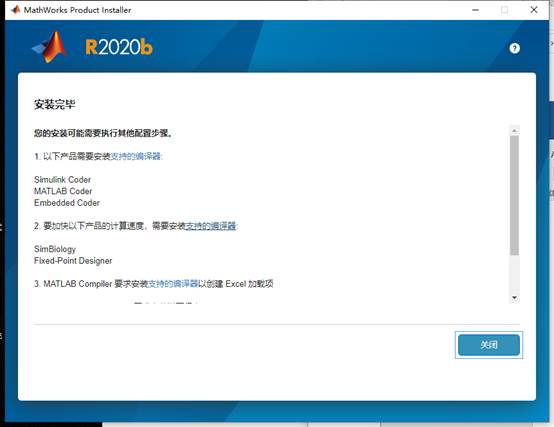11. 启动matlab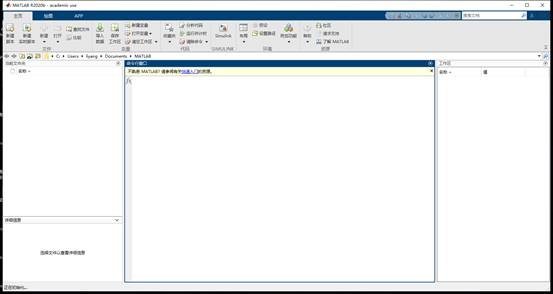l  MATLAB 入门之旅120 分钟需要登录）

l  Simulink 入门之旅 180 分钟 需安装 R2019a及以上版本）

l  机器学习入门之旅120 分钟需要登录）

l  Deep Learning 入门之旅120 分钟需要登录）

l  Stateflow 入门之旅180 分钟 需要登录）

l  图像处理入门之旅120 分钟需要登录）

l  10MATLAB进阶课程（需要登录）

l  MATLAB Online （网页版 MATLAB

l  MATLAB示例 （包含丰富的示例供教学和研究使用）a)       通过邮件联系 MathWorks 安装支持：

b)      通过电话联系 MathWorks 安装支持：

010-598270004

c)       在网上提交问题（需使用 MathWorks 账户登录）：

https://www.mathworks.cn/support/contact_us

d)      MATLAB 软件页面上提交问题：matlab软件校内的下载链接是：Matlab for MAC

1. 启动安装程序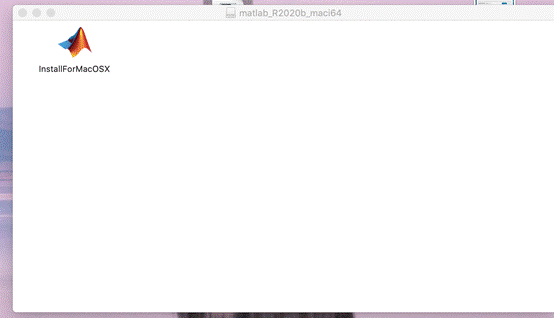2. 高级选项，我有文件安装密钥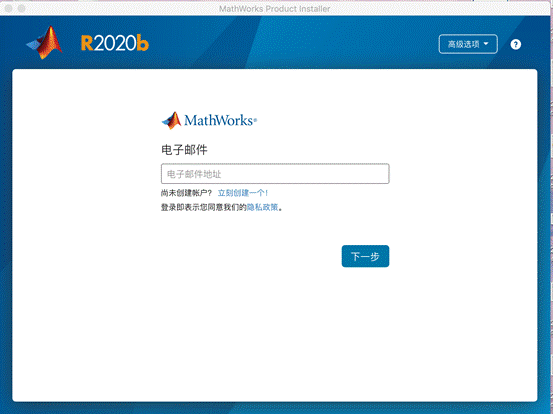3. 接受协议，下一步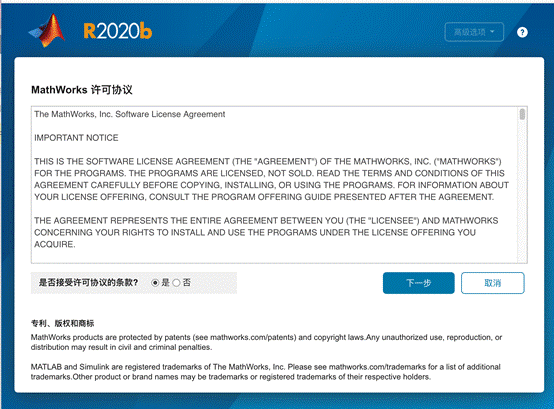4. 输入FIK 下一步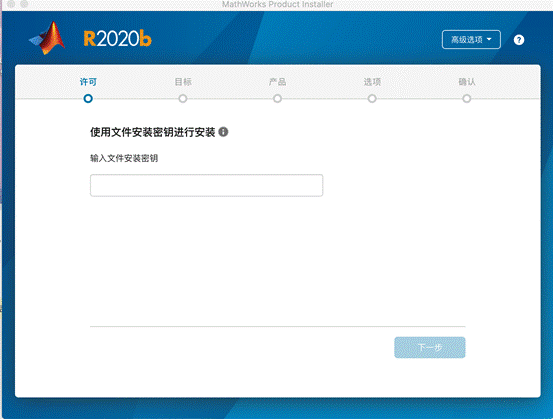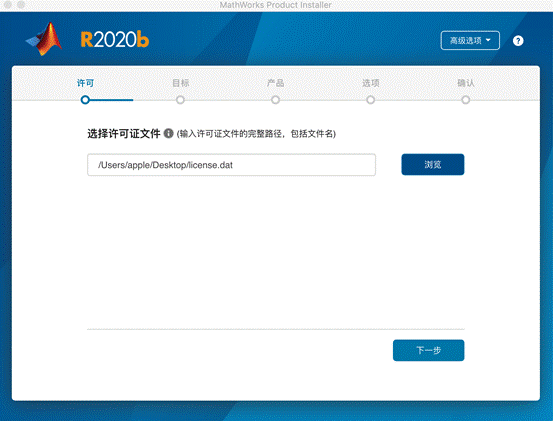6. 选择安装路径 下一步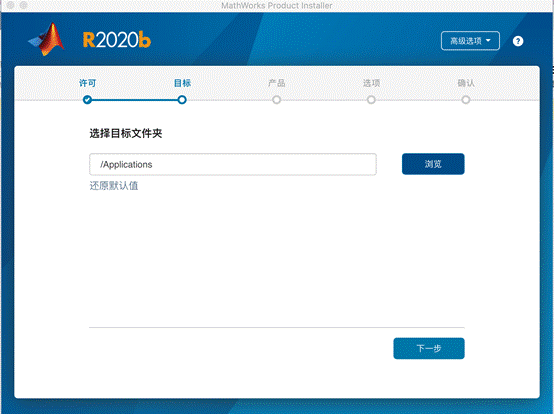7. 全选 下一步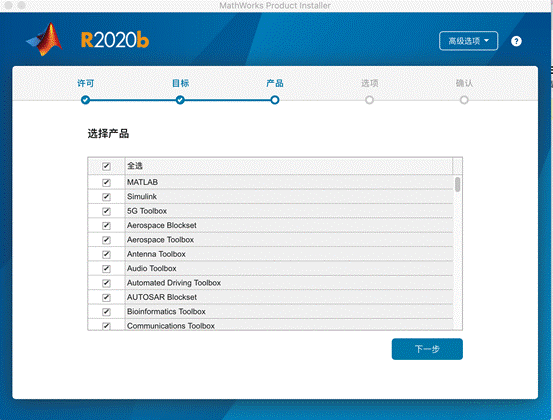8. 下一步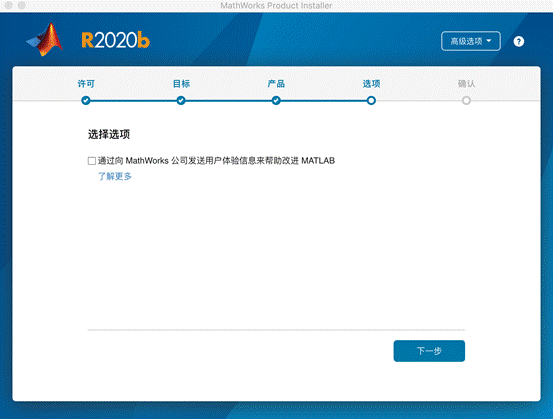9. 开始安装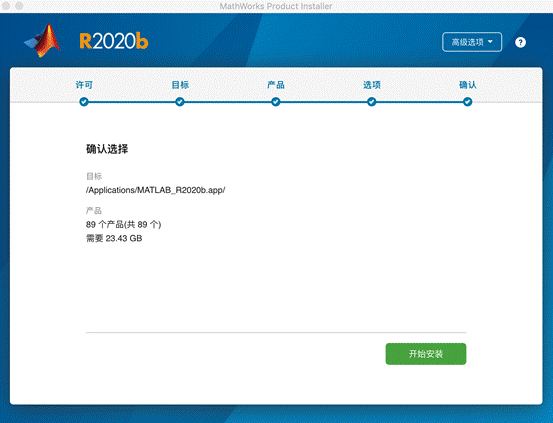10. 安装完成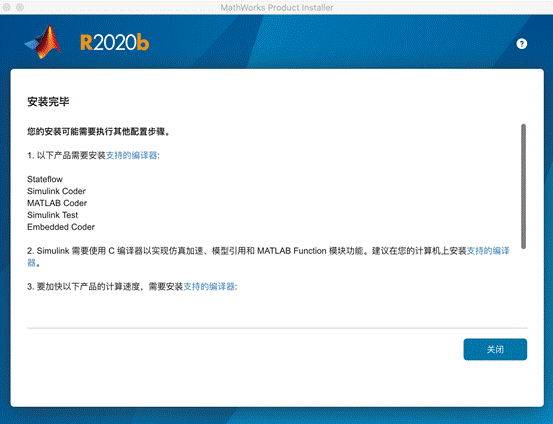11. 启动程序

matlab软件校内的下载链接是：Matlab for Linux

1. 先解压安装包到自定义目录 并在安装目录中执行以下命令，将打开安装页面。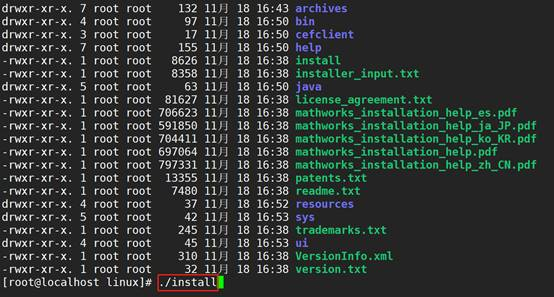2. 高级选项中，选择我有安装密钥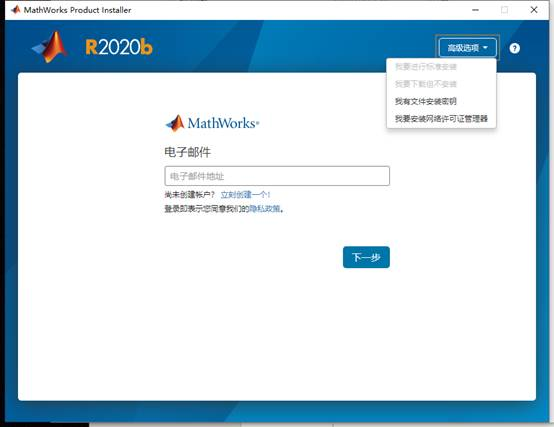3. 接受协议，下一步4. 输入FIK 下一步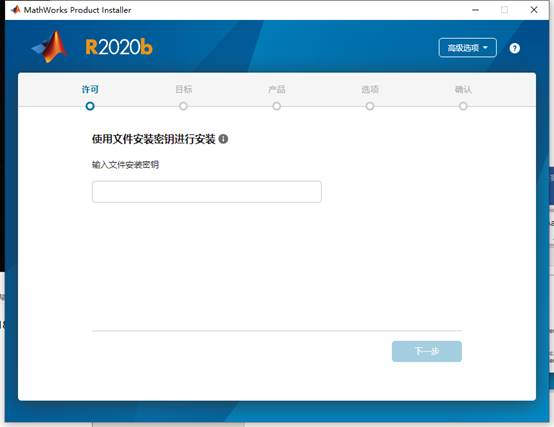6. 选择安装路径 下一步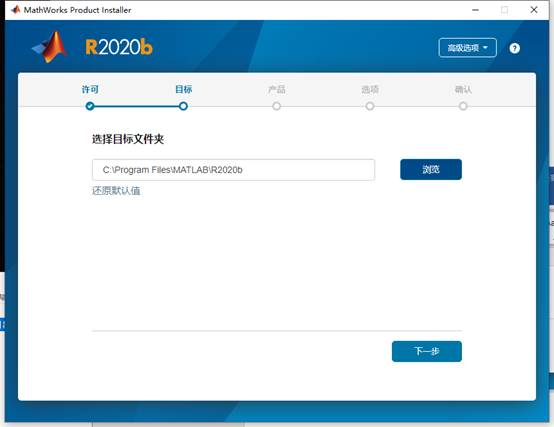7. 全选 下一步8. 创建桌面快捷方式 下一步9. 开始安装10. 安装完成11. 启动matlab